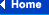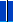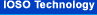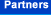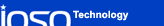## Robust Design Optimization Strategy of IOSO Technology

### Comparative Analysis of the Novel Strategy.

The paper presents the main capabilities of the Robust Design Optimization Strategy (RDOS) of the IOSO Technology (Indirect Optimization based on Self-Organization). The capabilities of RDOS software are demonstrated using examples of solving complex multidimensional (up to 200 design variables) problems. The examples utilize both single and multiobjective optimization problems. These example problems summarize more than ten years of using Robust Design Optimization for solving real-life problems in various scientific and technical fields. The paper presents the assembly of the newly developed efficient approaches for solving problems requiring Robust Design Optimization. These approaches employ technology of multilevel, multiobjective, and parallel optimization both separately and simultaneously. Efficiency of the Novel Strategy for Robust Design Optimization is compared to the other approaches.

#### The Main Features of the Robust Design Optimization Strategy

IOSO Technology implements the new evolutionary response surface strategy. This strategy differs significantly from both the traditional approaches of nonlinear programming and the traditional response surface methodology. Because of that IOSO Technology algorithms have higher efficiency, provide wider range of capabilities, and are practically insensitive with respect to the types of objective function and constraints: smooth, non-differentiable, stochastic, with multiple optima, with the portions of the design space where objective function and constraints could not be evaluated at all, with the objective function and constraints dependent on mixed variables, etc.

The concepts of Robust Design Optimization and Robust Optimal Control allow finding an optimal technical solution for the particular technology level, accounting that such a technical solution could be realized in practice with high probability. Some other approaches perform evaluation of probability parameters only after the deterministic optimal solution is found or employ very simplified estimates of probability parameters during optimization process. The distinctive feature of the Robust Design Optimization is that optimization problem is solved using stochastic formulation directly, when the evaluation of probability parameters is performed at each iteration. This results in reliable arrival to the truly robust optimal solution. High efficiency of the Robust Design Optimization is provided by the highly efficient capabilities of the developed stochastic optimization algorithms, that reliably work when high level of noise is present in responses. This is confirmed by the thorough testing of the algorithms using well-known test functions. Using our Robust Design Optimization concept results in considerable (several orders of magnitude) cost and time reduction when developing new highly efficient technical systems. Using Robust Design Optimization concept also provides the considerable (several times) risk reduction when new technical solutions are implemented. These features were demonstrated during optimal calibration of the actual automotive engine, when the time reduction of five times was achieved. When applying the Robust Design Optimization concept to design of the new axial compression the cost was reduced by more than two orders of magnitudes.

The distinctive feature of our approach is the ability to solve the problems with the large number of design variables (hundreds) and objectives (dozens). These features are demonstrated using the problem of geometry optimization of the actual axial compressor with 140 design variables when quasi 3-D CFD solutions were used for analysis.

### Multilevel Robust Design Optimization Strategy

The distinctive feature of the Multilevel Robust Design Optimization strategy is the use of mathematical models of various fidelity (from the lowest to the highest) during the solution process and adaptive switching between them. This procedure provides minimization of the number of times the high fidelity models are used without reducing the accuracy of the resulting solution. The efficiency of this procedure is demonstrated using the compressor optimization problem. This example demonstrates that it is possible to solve the optimization problem when the number of times the highest fidelity model is involved is less than the number of design variables. This provides considerable (several orders of magnitude) reduction in CPU time required for solution of complex optimization problems.

### Multiobjective Robust Design Optimization Strategy

It is shown that the developed algorithms of the Multiobjective Robust Design Optimization successfully solve the multiobjective (dozens of objective functions) multidimensional (hundreds of design variables) nonlinear optimization problems. The main advantages of the proposed algorithms over traditional mathematical programming strategies are the following.

• extreme accuracy approaches are not involved in solving multiobjective problems
• the algorithms determine the desired number of Pareto-optimal solutions, so that these solutions are uniformly distributed in the space of objective functions
• it is possible to solve the optimization problems where the objective functions exhibit complex topology: non-convex, non-differentiable, with many local optima
• flexible automatic adoptation of the internal mathematical models to the topologies of the objective functions and constraints
• high probability of locating a global optimum in a design space with many local optima
• relatively small number of high fidelity mathematical models evaluations
• it is possible to naturally employ the parallelization of the computational process

These advantages are the basis for the wide use of the proposed method in the real-life problems.

### Parallel Robust Design Optimization Strategy

The distinct feature of the Parallel Robust Design Optimization is the ability to reduce the solution CPU time faster than the increase in the number of processors used to solve the problem. For example, it is shown that using 20 processors it is possible to speed up the solution 40 times and more. High efficiency of our procedure is due to not only the simple and typical procedure of parallelizing computation of objective function and constraints as it is done in the majority of the known apporaches. In addition to that we parallelize the optimization process as a whole. This is accomplished by obtaining maximum possible information about the topology of the objective function and constraints using our specific response surface methodology at each iteration. Particularly, we construct a set of various approximation function with various properties (local and global accuracy, robustness, good prediction capabilities, etc.). Analysis of the set of these functions and defining new points for analysis at the next iteration is also done in parallel. It is shown that such approach provides considerable (several orders of magnitude) reduction in time required to solve optimization problem. Such approach also results in maximum usage of available computational resources, because the number of processors that could be efficiently employed is not dependent on the dimensionality of the problem. For example, for the problem with 10 design variables it is possible to efficiently use 10, 20, 100 and more processors.

### Comparative Analysis of the Novel Robust Design Optimization Strategy

The final part of the paper presents the results of the comparative analysis of various Robust Design Optimization strategies applied to test problems and real-life problems. The results show that due to use of these approaches the efficiency of the optimization process could be increased 5-10 times. This indicates that combining these procedures significantly broadens the capabilities of the Robust Design Optimization strategies when applied to real-life systems. This combination is also shown to be a powerful tool for finding new technical solutions, which in turn provide the maximum possible efficiency of complex systems.

##### Egorov, I.N., Kretinin, G.V. and Leshchenko, I.A. Sigma Technology, Moscow, Russia